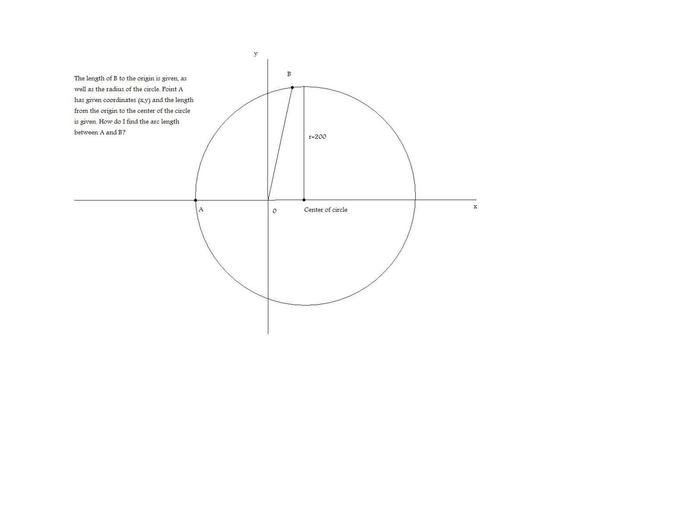# Arc Length

## Homework Statement## Homework Equations

The arc length equation?

## The Attempt at a Solution

I don't know where to begin.

Last edited:

## Answers and Replies

CompuChip
Science Advisor
Homework Helper
What is "the arc length equation", do you mean $L = r \theta$ (with $\theta$ in radians)? If so, yes, that is useful. r is given, how do you find $\theta$?

Also in your image you have drawn a vertical line stating r = 200. That line is not a radius of the circle, did you mean to put the label next to the line OB?

O (zero) is for origin and 200 is the radius, i just put the line there to indicate where the center of the circle is. The circle is not centered with the origin that is why, just shifted to the right.

CWatters
Science Advisor
Homework Helper
Gold Member
I would add two lines to the drawing...

1) From B to the center of the circle
2) From point B down to the x-axis.

Some triangles will be obvious.

I'd also mark the angle A-(center of circle)-B and call it θ

Then start with L = rθ and make substitutions. eg find a way to express θ in the required co-ordinates.

CompuChip
Science Advisor
Homework Helper
O (zero) is for origin and 200 is the radius, i just put the line there to indicate where the center of the circle is. The circle is not centered with the origin that is why, just shifted to the right.

I missed that, my bad.
Maybe it is slightly easier if you shift the center of the circle to the origin, which will make the coordinate of B (x - c, y) instead of (x, y), where (c, 0) is the center of the circle. Then, as I said, you will need to find $\theta$.

Thanks so much for your help guys, i found the answer :) Regarding the L=r theta, I actually found an easier way to calculate the arc length. If u divide the theta angle in degrees by 360 and put it equal to the L divided by the circumference it gives you the exact same answer. Like this, degree/360 = L/circumference and solve for L.